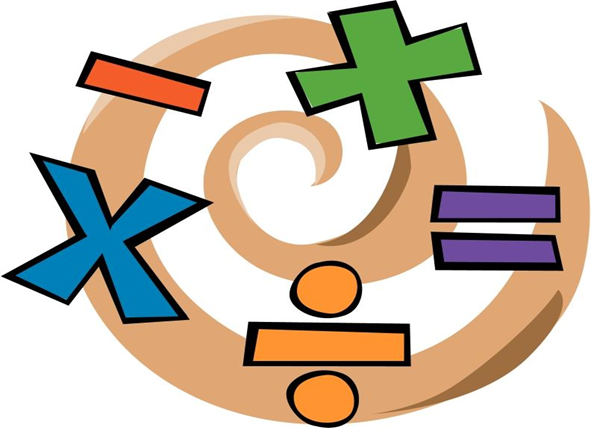•Course Description Math 8

Grade 8 Mathematics students build their understanding of mathematics by studying proportions, geometry, probability, statistics, order of operations, square roots, and other algebraic concepts. They will understand the concept of function as a rule that assigns to each input exactly one output. Students will translate among representations of functions and describe how aspects of functions are reflected in the different representations. Grade 8 Mathematics students will complete work on volume and surface area by investigating cones and cylinders and will solve real-world and mathematical problems involving measureable attributes of two-dimensional and three-dimensional objects. Students will understand the statement of the Pythagorean Theorem and its converse and explain why the Pythagorean Theorem works in terms of decomposing squares.

2016 SOL'S
First Nine Weeks 7.3 Integers  8.2 Subsets of Real Numbers 8.3ab Determine perfect square b.Find square root 8.1(b) Compare and Order  F, D, %, Sc. Not. 8.1(a) Simplify Expressions Order of Operations  8.3(a) Ratios and Proportions, Scale Drawings 8.3(a) Solve Practical Problems  Involving Percent, and Banking 8.3(b) Percent increase/decrease PERFORMANCE TASK-Corner Stone SOL8.3 8.4 Replacement Values
Second Nine Weeks 8.14(b) Properties and combine like terms 8.17Solve two-step equations with properties 8.18Solve two-step inequalities graph on number line One-Step Equations&  Inequalities.Two -Step Equations & Inequalities Variables on Both Sides 6.11Graphing on a Coordinate Plane 8.15 Identify domain, range,  independent and dependent variables 8.15 Relations and Functions 8.16 Graph Linear Equations in 2 Variables
Third Nine Weeks 8.9ab Pythagorean 8.5 Angle Relationships   8.10 Solve Area and Perimeter Problems  2-D Shapes Composite Figures 8.6 ab Volume and Surface Area describe how changing one attribute affects volume and surface area 8.8 Three dimensional models 8.7ab Transformations
Fourth Nine Weeks  8.11ab Probability  Performance task -Corner Stone SOL8.12abc Box Plots
8.13abc Scatterplots